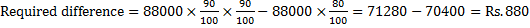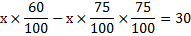# Profit and Loss for IBPS PO : Set 5

Q.1) A Grinder is listed at Rs.8000 and a discount of 15% is offered on the listed price. What additional discount must be offered to the customer to bring the net price to Rs.6120?

a) 15%

b) 12%

c) 9%

d) 10%

e) 20%

d

Selling price of the grinder=Rs.6120

8000× 85/100×x/100=6120

x=(6120×100×100)/(8000×85)=90

Discount =100-90=10%

Q.2) If the selling price with 20% profit on one article is the same as a selling price with 11.11% profit on another article, then what is cost price of the two articles?

a) Rs.250, Rs.300

b) Rs.280, Rs.290

c) Rs.200, Rs.250

d) Cannot be determined

e) None of these

e

Cost price cannot be determined from the given data.

Q.3) Jaleel bought a radio with 28% discount on the labelled price. Had he bought it with 32% discount, he would have saved Rs.160. What is the labelled price of the radio?

a) Rs.1600

b) Rs.3600

c) Rs.4000

d) Rs.4200

e) None of these

c

Let labelled price of the radio be x.

72/100 x-68/100 x=160

4/100 x=160

x=4000

Q.4) The marked price of a LCD T.V is Rs.75000. If successive discounts of 12%, 10% and 8% be allowed, then what is the selling price of the LCD T.V?

a) Rs.55768

b) Rs.54648

c) Rs.52168

d) Rs.58024

e) None of these

b

Selling price=75000×88/100×90/100×92/100=Rs.54648

Q.5) What is the difference between selling prices of refrigerator with discount of 20% to two successive discount of 10% each, if the marked price of refrigerator is Rs.88000?

a) Rs.800

b) Rs.820

c) RS.880

d) Rs.840

e) None of these

cQ.6) If the difference between the selling price with a discount of 40% and two successive discounts of 25% on a marked price of a fan is Rs.30, then what is the marked price of the fan?

a) Rs.750

b) Rs.700

c) Rs.850

d) Rs.800

e) None of these

d

Let marked price of the fan be x.0.6x-0.5625x=30

x= 30/0.0375=800

Q.7) A shopkeeper sells two shirts for Rs.1500 each. On one he gets 20% profit and on the other 20% loss, What is his profit or loss in the entire transaction?

a) 4% profit

b) 4% loss

c) 5% loss

d) 5% profit

e) No profit or loss

b

% Loss=((loss/gain%)/10)^2

=(20/10)^2

=2^2

=4% loss

Q.8) A man sells two helmet for Rs.1200 each, neither losing nor gaining in the deal. If he sold one helmet at a loss of 20%, the other helmet is sold at a gain of what percent?

a) 33 1/3 %

b) 30 1/6%

c) 16 1/6%

d) 20%

e) None of these

a

Total S.P. = Rs.2400

Total C.P. = Rs.2400

S.P. of 1st helmet = Rs.1200 (at 20% loss)

C.P. of 1st helmet=1200/80×100=1500

C.P. of 2nd helmet =2400-1500=900

S.P. of 2nd helmet = Rs.1200

% Gain on 2nd helmet=(1200-900)/900×100=300/900×100=331/3%

Q.49) A laptop and a mobile phone were sold for Rs.30000 each. In this transaction, the laptop sold at 25% loss whereas the mobile phone sold at 20% profit. What is the overall profit/loss in the transaction?

a) 6 7/13% profit

b) 7 6/13% loss

c) 7 2/7% profit

d) 7 9/13% loss

e) None of these

d

Total selling price=60000

Cost price of laptop=30000/75×100=40000

Cost price of mobile phone=30000/120×100=25000

Total cost price=40000+25000=65000

% loss=(65000-60000)/65000×100=79/13%

Q.50) Ragavi purchased 40 chairs at a price of Rs.250 per chair. He sold 12 chairs at profit of Rs.20 per chair and 10 chairs at a loss of Rs.15 per chair and the remaining chairs were sold at a profit of Rs.10 per chair. What is the average profit per chair (Approximately)?

a) Rs.12

b) Rs.15

c) Rs.5

d) Rs.7

e) Rs.9

d

Cost price of 40 chairs=40×250=10000

Selling price of 40 chairs= (12×270) + (10×235)+(18×260)

=3240+2350+4680=10270

Profit= 10270-10000=270

Average profit per chair=270/40≅Rs.7